# Errors in the Steady State and Quasi-SS Models

The Steady State model of the Universe was proposed in 1948 by Bondi and Gold and by Hoyle. Bondi and Gold adopted the "Perfect Cosmological Principle", and added the assumption that the Universe was the same at all times to homogeneity (the same in all places) and isotropy (the same in all directions). The Universe is observed to be expanding, so if the density remains the same, matter must be continuously created. This radical assumption is not the reason that the Steady State model is now rejected. Like any good scientific model, the Steady State made many quantitative testable predictions, and these predictions inspired many observational campaigns. As a result of these observations it became clear that the Steady State model predictions were not correct.

At the time the Steady State model was proposed, the Big Bang model was in trouble because the value of the Hubble constant was clearly bigger than the inverse of the age of the Universe. If the Universe is the same at all times, the value of the Hubble constant must really be constant, so v = dD/dt = HD has an exponential solution and the scale factor varies like

```a(t) = exp(H(t-to))
```
Furthermore, since the radius of curvature of the Universe can not change, but must expand, the radius has to be infinite. Thus the Steady State model has flat spatial sections like the critical density Big Bang model. Since the expansion of the Universe spreads the existing matter over a larger and larger volume, but the density stays constant, the Steady State model requires continuous creation of matter. The average age of matter in the Steady State model is <t> = 1/(3*Ho) but some galaxies are much older than the average, so the age of the globular clusters can be accommodated if the Milky Way is older than the average. The space-time diagram below shows the Steady State model:The past light cone of the central galaxy ("us") is shown in red. Note the continual creation of galaxies so the average density remains the same.

The Steady State model makes some definite predictions. The first one to be tested involved the number of faint radio sources. In the 1950's astronomers found that radio sources were typically much more distant than typical optical galaxies, so modifications to the usual source count law due to cosmology were expected. For the standard Big Bang model the counts were expected to fall below the usual "8 times more sources for 4 times fainter limit" law by an amount given approximately by 1/(1+z)4 where z is the redshift of the sources. This law assumes that radio sources are conserved, so a given section of the Universe has the same number of radio sources at all times. Because the volume of the section was smaller by a factor of (1+z)3 at early times, the actual density of radio sources was higher by a factor of (1+z)3. The density was constant in the Steady State model, of course, so the count correction factor would be given by 1/(1+z)7. The diagram below shows what was expected and actually seen: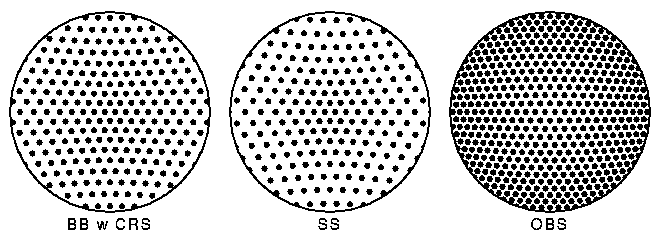The Big Bang should have a deficit of faint sources, the Steady State should have an even bigger deficit, but the observations showed a surplus of faint sources. The Steady State model has no adjustable parameters to correct for this error, but the Big Bang does. The assumption of conserved radio sources (CRS) can be dropped in favor of an excess of radio sources 1-3 Gyr after the Big Bang. Thus the Steady State failed the radio source count test, while the Big Bang passed by "winning ugly" - introducing a new parameter to describe a new datum. See Maran's review of Hoyle's book, Galaxies, Nuclei, and Quasars. Maran describes the birth and death of the Steady State theory without reference to the microwave background.The Big Bang was originally proposed in the context of making all the elements. But the lack of a stable nucleus with atomic weight A=5 meant that only isotopes of hydrogen, helium and a trace of lithium are produced in Big Bang Nucleosynthesis. In the original Steady State proposal, all of the heavy elements were produced in stars by burning hydrogen into helium and then combining several helium nuclei [alpha particles] into heavier nuclei like carbon (3 alpha particles) and oxygen (4 alpha particles). In general the heavy element abundances relative to hydrogen are proportional to each other. Some stars have very little oxygen and these usually also have very little iron, and so on. But helium is definitely an exception to this rule. There is a non-zero floor to the helium abundance as the oxygen abundance goes to zero. This is shown in the plot at right which shows the helium and oxygen abundances relative to hydrogen by number of nuclei in the Sun and several ionized hydrogen nebulae [H II regions] in our Milky Way [M42 is the Orion nebula, M17 is the Omega nebula], in the nearby dwarf galaxies known as the Large and Small Magellanic clouds [LMC and SMC], and in other extragalactic H II regions. This plot clearly shows that solid line, which allows for the primordial helium produced in the Big Bang, is a much better fit than the dashed line which is the prediction of the Steady State model with no primordial helium. The data for this plot were taken from Figure 1b of a recent paper on the element abundances in the Sun. Shortly before the discovery of the CMB killed the Steady State model, Hoyle & Tayler (1964, Nature, 203, 1008) wrote "The Mystery of the Cosmic Helium Abundance" in which they decided that most of the helium in the Universe was not produced in stars. Hoyle held open the possibility of explosions in supermassive objects instead of a single Big Bang, but ordinary stars were ruled out.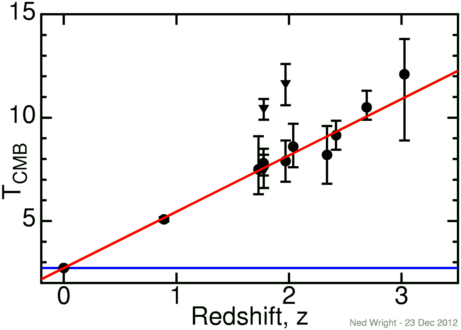The discovery of the cosmic background blackbody radiation came later, and completed the death of the Steady State. The Universe now is not producing a blackbody since it is not isothermal and it is transparent instead of opaque. In the Steady State the Universe was always the same so it never produced a blackbody. Hence the existence of a blackbody background ruled out the Steady State. In addition, the temperature of the cosmic background can be measured in some very distant clouds that produce absorption lines in the spectra of quasars. The neutral carbon atoms in these clouds are excited to an excitation temperature that can be measured using line ratios. These excitation temperatures are upper limits to the CMB temperature and are shown as triangular data points at right. In some clouds corrections for other sources of excitation can be made, giving a direct measure of TCMB, shown as a round data point. This data agrees very well with the evolution expected in the Big Bang model: TCMB = To(1+z), which is shown as the red line in the figure. Even if there were some unknown mechanism for producing a blackbody radiation field in the Steady State model, its temperature would have to be constant as a function of redshift as shown by the blue line and these observations reject this model. Noterdaeme et al. (2010) give several of the points in the plot and find that TCMB(z) agrees very well with the Big Bang prediction, but differs from the Steady State prediction by 37 standard deviations. Saro et al. use South Pole Telescope observations of the Sunyaev-Zeldovich effect cross-over frequency for cluster of galaxies versus their redshifts. to find that TCMB = To(1+z)1-α with α = 0.017 ± 0.029 which is 32 standard deviations away from the Steady State prediction of α = 1. Hurier et al. used Planck data to get α = 0.009 ± 0.017 which is 58 standard deviations away from the Steady State prediction.

The Quasi-Steady State Cosmology is an attempt by Hoyle, Burbidge and Narlikar to allow for the evolution of the CMB temperature and to explain the surplus of faint radio sources in a Universe that is always the same over the very long term. A sinusoidal pulsation is superimposed over the exponential growth of the scale factor a(t), giving the space-time diagram below.During the previous large phase of the Universe, our past light cone (in red) was very large, and this gives a large number of faint sources. Unfortunately for Hoyle, Burbidge and Narlikar these sources are blueshifted -- as indicated by the blue tint on the space-time diagram, and NO faint radio source has ever been observed to have a blueshift. This data disproving the QSSC model existed before Hoyle, Burbidge and Narlikar published -- so the QSSC model was definitely an error by formerly great cosmologists.

## The NEW QSSCHoyle, Burbidge and Narlikar have not abandoned the QSSC but have continued to develop it. In recent papers they have presented a new version of the QSSC that has a greater connection to standard physics. In this model, there is a creation field that gives an energy density that is negative and scales like radiation. This negative energy density becomes dominant at high redshifts and causes the bounce in the QSSC. The recollapse that leads to the periodic nature of the QSSC is caused by a negative vacuum energy density. As a result the evolution of the scale factor is no longer a sinusoid modulated by an exponential [the red dashed curve at right], but rather a considerably more cuspy function shown in blue.

Some of what follows will be fairly technical, since many astronomers will not want to spend the time needed to understand what the QSSC is saying. Given that the expansion rate of the Universe goes to zero at amin and amax, and that the curvature is zero, one can easily solve for all three of the relevant densities, finding for zmax=5 and amax/amin=(1+0.811)/(1-0.811) that Omegavac = -0.358, Omegam = 1.623, and Omegarad = -0.271. These parameters thus give a deceleration parameter qo = 1.623/2+0.358-2*0.271 = 0.63.Given that the deceleration parameter is close to the Einstein-de Sitter value of qo = 0.5, it is not surprising that evolution of the scale factor a(t) from the last minimum until now follows the EdS curve quite closely. The figure at right shows the EdS curve in red and the Steady State curve in blue.

Note that the new QSSC is accelerating only during the bounce. At other times it is decelerating. Also note that the matter density in the QSSC is about 5 times larger than most current estimates.

The units for the time axis in the figure are 1/Ho, so the time since the last bounce for the QSSC with the chosen parameters is almost exactly the same as the age of the EdS Universe: Hot = 2/3.If the QSSC is decelerating instead of accelerating, how is it that Banerjee et al. (2000, AJ, 119, 2583) claim to be able to fit the distant supernova data that is evidence for an accelerating expansion? The answer lies in extinction by the carbon and iron whiskers that the QSSC uses to convert star light into CMB photons. Since the QSSC has a larger deceleration than the EdS model, it requires much more gray dust than the open model and slightly more than the EdS model considered by Aguirre (1999, ApJL, 512, L19). The figure at right shows the distance modulus relative to c/Ho, DM = 5 log10(DL Ho/c), plotted vs redshift for the models shown in the previous figure. The red curve is the EdS model, while the black curve is the QSSC without absorption. The blue curve is for the Steady State model. The magenta curve is for the best fit OmegaM=0.3 vacuum dominated flat model. The green curve is the QSSC model with one magnitude of extinction per Hubble radius locally. The green curve crosses the magenta curve at z = 0.45, so it will fit the supernova data quite well. But Aguirre and Haiman (2000, ApJ, 532, 28) find that the amount of dust needed to go from the EdS model to the supernova observations is not allowed by the Cosmic Infrared Background data, so the slightly greater amount of dust needed by the QSSC would also be ruled out.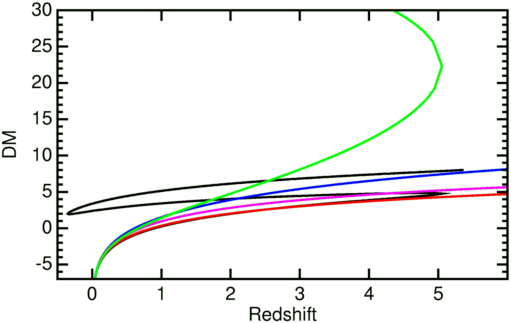At larger redshifts the extinction grows quite rapidly. Note that Equation (30) of Banerjee et al. which gives the extinction as a function of redshift has a serious error which greatly affects the answer at high redshifts. But using the corrected equation gives the DM vs z curves shown in the figure at right. The black curve, the QSSC without extinction, shows the loop back to low DM [brighter sources] during the previous maximum of a(t) that the old QSSC used to explain the excess of faint radio sources. But with the amount of dust required to fit the supernova data, there is so much extinction through the minimum size epoch that it becomes impossible to see anything prior to the minimum.

In the QSSC, the dust opacity in the millimeter waveband is higher than the optical opacity, so the Universe would have to be optically thick at z=0.3 for CMB radiation. But the recent preprint by Narlikar et al., which miscalculates the small angular scale CMB anisotropies, assumes the Universe is transparent up to zmax. So if Narlikar et al. is right, then Banerjee et al. must be wrong, and vice-versa. These papers cannot both be right, since Banerjee et al. requires a high opacity while Narlikar et al. requires a low opacity. But actually both these papers are wrong. The Narlikar et al. model should give a small-scale anisotropy in the CMB that is the Fourier transform of the two-point correlation function of galaxies. But the two-point correlation function of galaxies is a power law, so the angular power spectrum of the CMB would be a power law, and not have a peak. Narlikar et al. just assert there would be a peak without any reference or justification.

In 2002, Narlikar et al. again present an "Interpretation of the Accelerating Universe" that requires a large opacity, while Narlikar et al. presents an interpretation of the CMB anisotropy that requires a low opacity. These articles were submitted to different journals, and refer to each other as successful calculations of the QSSC model, but they in fact contradict each other. Presumably this is a deliberate attempt to deceive the casual reader, since Narlikar et al. should know what Narlikar et al. is doing.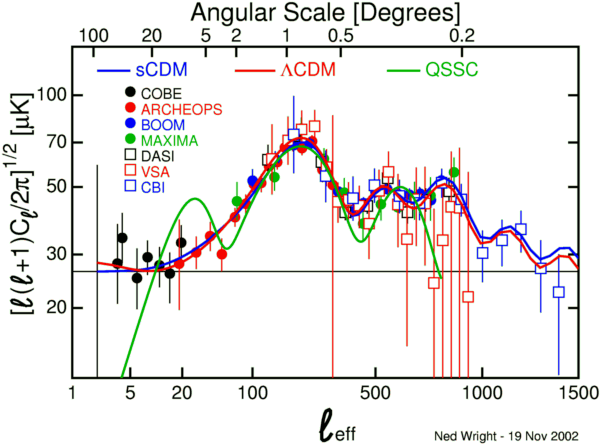The claim by Narlikar et al. (2003, ApJ, 585, 1) to fit the CMB anisotropy data is false as well. The graph above shows the pre-WMAP compilation of CMB data, along with red and blue curves which are versions of the cold dark matter dominated models with different parameters, and the solid curve from Figure 4 of Narlikar et al. This model obviously does not fit the COBE data which were published in 1992. Narlikar et al. hide this discrepancy by only plotting binned CMB data.

The claim by Narlikar, Burbidge and Vishwakarma (2007, J. Astr. & Ap., 28, 67) to fit the CMB anisotropy data is also false. To make this claim Narlikar made a poorly justified change in his 2003 model to better fit the data. But since the motivation for the model was rather ad hoc to start, it is pointless to complain about an unjustified change. Narlikar et al. noted that their model did not fit the high ell data very well, but noted that these points changed quite a bit between the first year WMAP data and the three year WMAP data. However, the failure of the model to fit the high ell points in the three-year WMAP power spectrum is not because the high ell data had not settled down, but is rather a failure of the model at high ell, that can be seen better by fitting to a combined dataset with both WMAP data and data from the ground-based and balloon-borne experiments that have smaller beam sizes and work better at high ell.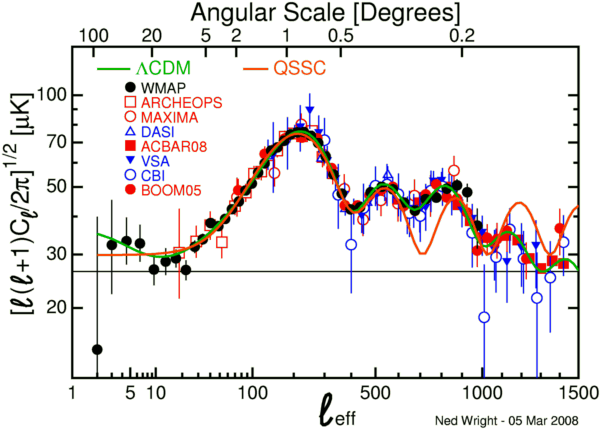The plot above shows this fit: the ΛCDM model in green fits all the data very well, while the QSSC model in orange fits rather poorly. There is a difference in χ2 of 516.3 between the two models, which both have 6 free parameters. Narlikar et al. chose the CMB angular power spectrum as the one and only plot in their paper, but their model does not fit the WMAP three year data nor does it fit the CBI and ACBAR data that were already published. It is very clear that the QSSC CMB angular power spectrum model proposed by Narlikar et al. does not fit the CMB data.

The nucleosynthesis theory in the QSSC leading to the standard helium abundance is frozen in the 1960's, based on the eight-fold way or flavor SU(3). For some reason only up, down and strange quarks are produced. The suppression of flavor changing neutral currents means that all the strange quarks decay to up quarks, leading to a large excess of protons over neutrons. But if one allows for charmed quarks, or for all six quark flavors, then this proton excess goes away, and one gets the wrong ratio of H to He in the final products.